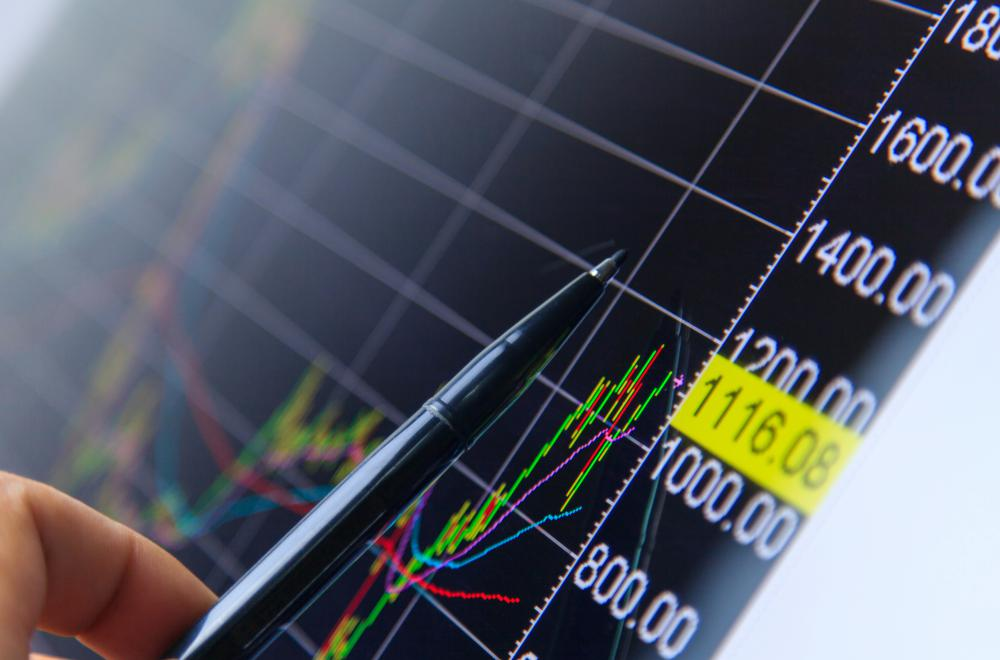# How do I Choose the Best Bond Yield?

Kristie Lorette

A bond yield is the amount of return on your investment or amount of interest earned on a bond that you invest your money in. High yield bonds return a higher return on the investment than those with lower interest rates or yields. Part of choosing the best bond yield is to calculate the yield of the bond, which considers the price paid to buy the bond, the face value of the bond and the interest payment of the bond.Bond traders must remain aware of the market’s shifts and must keep a vigilant watch over major jumps or dips in interest rates and payout structures.

To calculate the yield on a bond, divide the face value or coupon rate of the bond by the price you pay to buy the bond. For example, a bond issued at face value of \$1,000 US Dollars (USD) that has a 10% coupon rate yields \$100 USD in interest. When the same bond, with a face value of \$1,000 USD, sells at a discount rate of \$800 USD, then the yield on the bond increases to 12.5%.

Adversely, a bond with a face value of \$1,000 USD that sells at a premium of \$1,200 USD pushes the bond yield down to 8.33%. So, a major part of choosing the best bond yield is to evaluate whether you are paying face value, a discount or premium price to buy the bind in the first place.

The second major calculation is to calculate the yield to maturity (YTM). Unfortunately, this calculation is not a simple equation. Calculating this figure requires you to use a bond yield to maturity calculator, which you can find online, or use a financial calculator to input the figures. The answer to this calculation, however, tells you how much money you will earn on your investment in the bond if you hold it until the maturity date on the bond.

In short, however, if you understand how bonds behave, you can choose the best bond yield. Essentially, as bond prices go up, the bond yield goes down. When bond prices go down, then the bond yield goes up. This means that if you have the opportunity to buy a bond at a discount price, then you will yield more in the long-run than if you pay a premium or face value.

If you buy the bond at a premium price, then you will earn less of a yield than if you purchase it at face value or at a discount. If you buy the bond at face value, then you fall right in the middle — not earning as much as if you buy the bond at a discount, but earning more than buying the bond at a premium.

## You might also Like